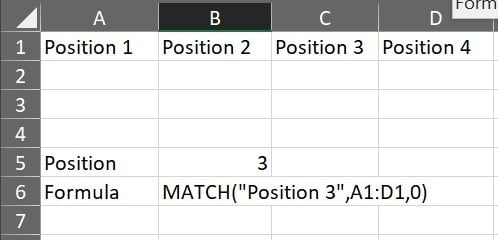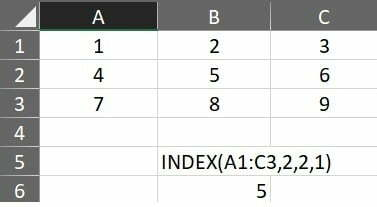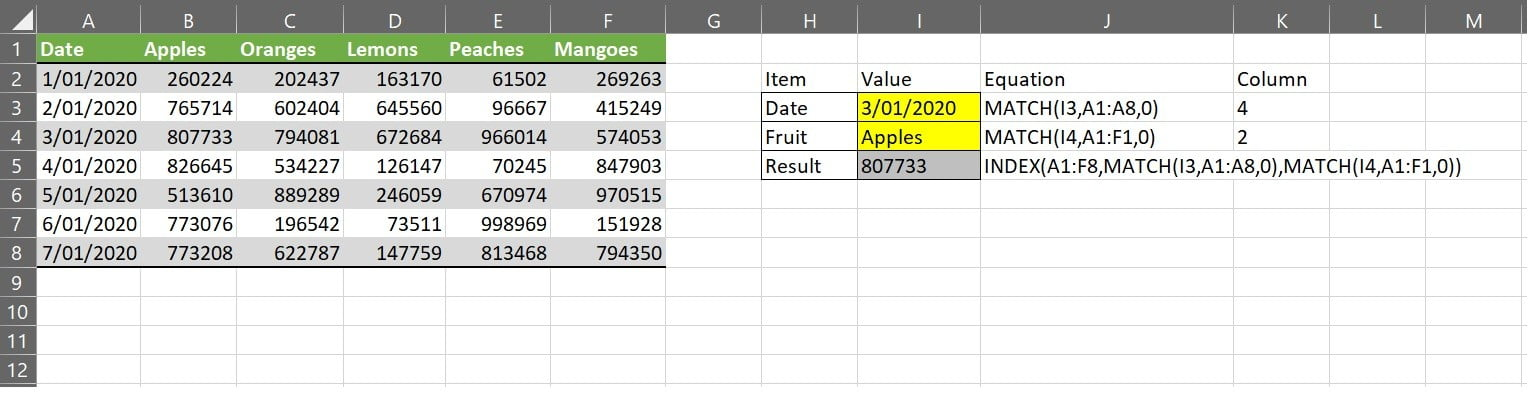Right click has been disabled on this site.

# Excel – Index & MatchExcel is an extremely powerful tool for data manipulation and one that I have to use on a regular basis. As part of this I have to often work with a range of different data layouts which need to be manipulated to suit ingestion into a different system (e.g. database).

With that in mind, I thought it would be beneficial (even for myself) to start to note down some of the various formula's and function's which allow me to manipulate the data.

In this post I am going to focus on two closely linked formula's; Match & Index. We will start with match, then onto index and finally show how these two can be used in a combined formula.

## Match

Match allows you to query a range of data for a specific value, and then to return the row / column number (e.g. index) in which that data was obtained. The formula is as per the below;

=Match(lookup_value, lookup_array, match type)

lookup_value refers to the value you wish to match or find.

lookup_array refers to the range of cells or array reference you want to search in.

match_type (optional) refers to how to match the data, 1= exact match or next smallest, 0 = exact match, -1 = exact match or next largest.

I have used the match in the below example, in this case I want to determine which position the text “Position 3” is located in. In order to achieve this I have used the formula =MATCH(“Position 3”,A1:D1,0). Lets break this formula down;

Match(text to search for, range or array to search in, match type)

In the formula above, I am effectively saying search for “Position 3” in the cell range A1 to D1, and when you find an exact match let me know the position.The output of this formula is 3, this is basically saying that if the text was found in position 3 of the array / range, where the start position was A1. Since this is a horizontal range it would mean that the phrase “Position 3” is found at C1.

The same formula can also work vertically, in which case instead of looking at the column as the index we would be looking at the row number.

## Index

Index allows you to return a value at a given position within an index or array. These returned values can take the form of individual values, a well as entire rows or columns. The formula for Index is as per the below;

=INDEX(array,row_number,column_number,area_number)

Array refers to the range of cells you want to query.

row_number refers to the position of the row within that array

column_number refers to the position of the column within that array

area number refers to the range of value(s) you want to return.

Using the above formula, we could use index to get a value from within a table. Imagine we have a 3 x 3 grid as per the image below, and we want to get a value from the second row and second column. To achieve this we can simply use the formula =INDEX(A1:C3,2,2,1).

This formula is effectively saying I want you to look in the range A1 to C3, and return one value, where that value is from the second row and second column.The output of this specific formula is five. Because two columns (including the first column) puts the cell in the B column, and two rows (including the first row) puts the cell in the row 2, therefore the cell being referenced is C2 with a value of five.

It should be noted, that in this case we have hardcoded the index function however, the power of index is extended much more when you are using it dynamically (e.g. Match and Index).

## Combing Match and Index.

We have previously talked about how we can use match to find the relative position in which a specific criteria is found (e.g. where do I find the phrase “Position 3”, and we have also shown how we can use Index to return a specific value but this becomes far more powerful when we combine these toghether.

Imagine the scenario below, we have a table which contains a range of date(s) along the “A” column, with different fruits being listed across the “1” row. This table represents fictional fruit sales for a wholesaler for a given date.Say we want a way to easily be able to query the table (which could be significantly bigger) for the sales of a particular fruit on a specific date, we could use Match to locate the Fruit and Date position, and index to return that value.

In the example above, the first step is to determine which row the date we are searching for falls within. In this case we are looking for the date “3/01/2020” and to find this we are using the formula =MATCH(I3,A1:A8,0). This is saying I want you to look for the value in cell I3 (3/01/2020) in the range A1 to A8, and return the relative position of this exact value (which is position A4, i.e. 4 rows from the start including the start row which is A1).

The second step is to determine which column the fruit we are searching for is within. In the case of the spreadsheet above we are looking for “Apples” and to find this we use the formula =MATCH(I4,A1:F1,0). This is saying I want you to look for the value in cell I4 (Apples) in the range A1 to F1, and return the relative position of this exact value (which is in position B1, i.e. two columns from the start including the start column which is A1).

The final step is to then use INDEX to return the value for these two matches. We could have a cell for each match position similar to what I have done above (e.g. K3 & K4) and the Index function would look like the below;

=INDEX(A1:F8, K3, K4,1)

This would look in the range of A1 to F8, and fetch the row value which is stored in K3 (e.g. 4) and the column value which we have stored in K4 (e.g. 2), it would then take one value from the table where the position would equate to four rows down from the starting point, and two columns across from the starting point (e.g. B4) which equals 807733.

Now this is all well and good, but you can also nest your functions, so instead of storing the row and column positions from the match formula we can just use these within the formula itself. In this case our consolidated formula would look like the below;

=INDEX(A1:F8,MATCH(I3,A1:A8,0),MATCH(I4,A1:F1,0),1)

In the above formula we are basically saying, I want you to return one value, from the range of A1 to F8, where;

• The row is the position you find the phrase in I3 within the range of A1 to A8 and;
• The column is the position you find the phrase in I4 within the range of A1:F1)

It is important to note, that the values returned are relative so you need to make sure that your ranges are matched, or that you consider if there is any offset.